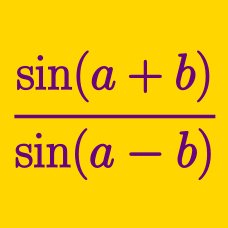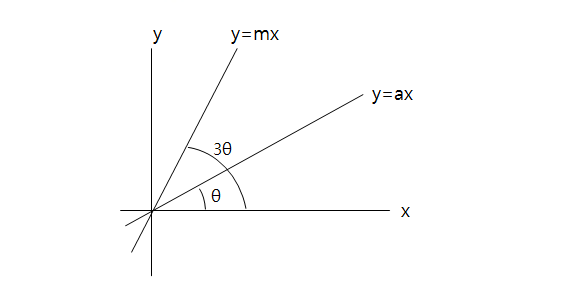Geometry

# Triple Angle IdentitiesIn the above diagram, the angle between $$y=ax$$ and the $$x$$-axis is $$\theta$$ and the angle between $$y=mx$$ and the $$x$$-axis is $$3\theta.$$ If $$a = \frac{1}{6},$$ what is the value of $$m?$$

If $$\sin \theta = \frac{1}{3},$$ what is $$\sin 3\theta?$$

What is $$6\times\left(\frac{3\times\tan10^{\circ}-\tan^3 10^{\circ}}{1-3\times\tan^2 10^{\circ}}\right)^2?$$

If $$\frac{\sin3\theta}{\cos3\theta+2\cos\theta}=7,$$ what is the value of $$\tan\theta?$$

If $$\frac{\sin3\theta}{\cos3\theta+2\cos\theta}=4,$$ what is the value of $$\tan\theta?$$

×